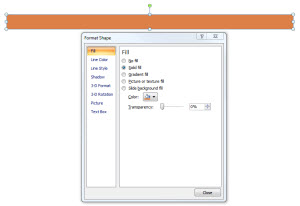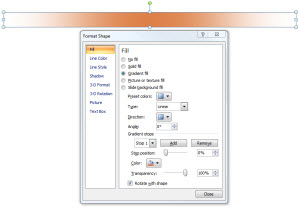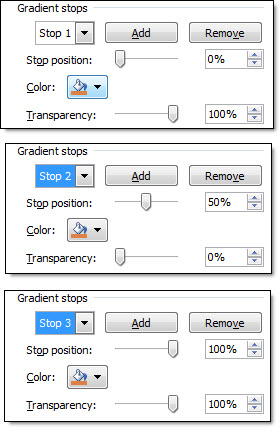Categories

# Gradient Visual Dividers

Lots of slides have a few main points, like this example:

I like to add a simple divider line as a visual break between each line. And I create it in PowerPoint using a simple gradient fill. So my sample slide looks like this:

To create the…

Lots of slides have a few main points, like this example:I like to add a simple divider line as a visual break between each line. And I create it in PowerPoint using a simple gradient fill. So my sample slide looks like this:To create the divider line, start with added a basic rectangle – no outline and the fill color you want.Then change to a Gradient Fill.Creating the feathered edge is a bit tricky:
1. Type = Linear
2. Angle = 0
3. Stop 1: position = 0, color = your pick, transparency = 0
4. Stop 2: position = 50, color = your pick, transparency = 100
5. Stop 3: position = 100, color = your pick, transparency = 100
** Delete any additional stops (only want 3)Last, adjust the rectangle height to be smallest possible.

Done!

– Troy @ TLC